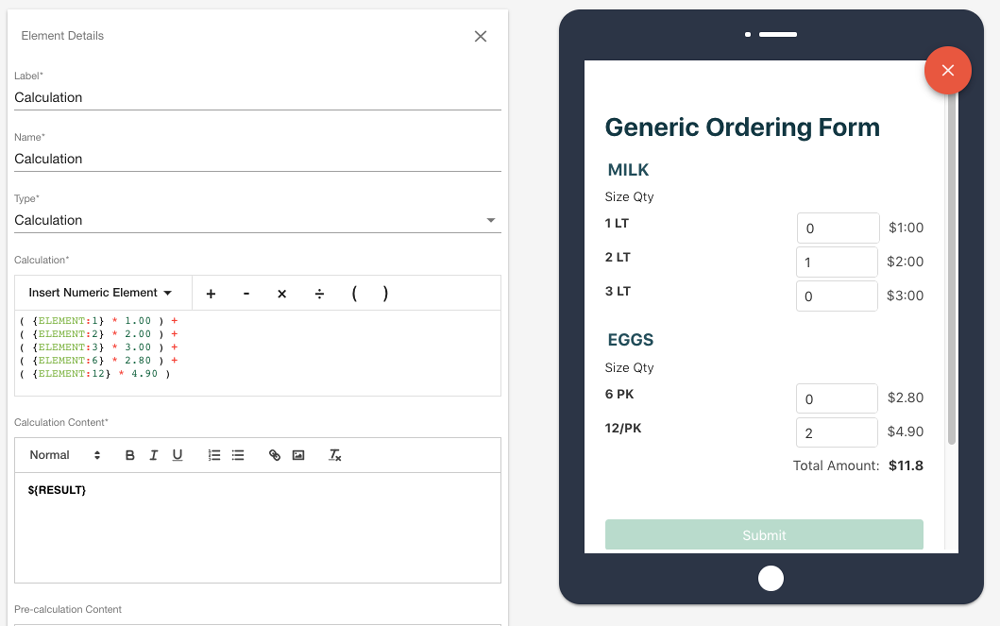Calculation Element

This element allows you to take the input from other elements and create calculations based on those inputs.

Calculations can be performed on both number, calculation, radio button, checkboxes and single select elements.

The calculation element has 3 separate inputs, Calculation, Calculation content, and Pre-calculation Content.

For a more hands on experience with calculations check out this interactive form.

Calculation

This is where the calculation for the element will be done. You are able to insert the element by using the Insert Numeric Element button.
This will give you a drop down to choose between your numeric elements.

These elements are represented as such {ELEMENT:element_name} where element_name will be the name of the element you select.

You are also given a selection of expressions (+ - / * ) you can use for the calculation, these are displayed in red.

Rounding

You are also able to round your inputs using the ROUND() function.

For example if you wanted to round the input of your currency element to 2 decimal places you would use:

ROUND({ELEMENT:element_name}, 2)

Null Values

You are able to have calculations with Null Values. Meaning the calculation can run without the need for all the calculation elements to have an input. This can be done using the ISNULL function similar to the ROUND function.

Include the ELEMENT in the {} curly brackets and after the comma add the number you want to use for when the element does not have a value. In the example below the element is treated as having a value of 0 until the user updates the element with an actual value.

ISNULL({ELEMENT:element_name}, 0)

Calculation Content

This is what will be displayed once the calculation has been completed. {RESULT} is where the result of the calculation will appear.
You are able to add other text around the result to help add context.

Pre-calculation Content

This is what will be displayed before the calculation is completed. This can be used to create place holders for your results.

Single Select elements and Radio buttons are able to be used as in calculation elements by using the value of the select or radio option.These are the values that will be used in the calculation element when the radio button is selected.

Let's see how this works in practice.

You'll notice that not all ratings have the same effect on the score.

'Story Telling', and 'Creativity and Originality' have much more of an effect on the score than 'Cinematography' and 'Editing'.

This is due to the value placed on those radio buttons. The value of the 'Engagement' (%20) is double that of the 'Cinematography' (%10) because of how the score is calculated. This can be done through setting appropriate variable, or set in the calculation itself.Calculation Examples

Here are some simple examples for how the calculation element can be used.

Example 1 (1+2)

Here you can see the Calculation taking in two number elements, ‘Number_1’ and ‘Number_2’ and adding the two numbers together.

For the Calculation Content we have added some helpful text before the {RESULT} to explain what is happening in the calculation.

This is an optional step the only thing that needs to be displayed here is the result.

In the Pre-calculation Content you can see we have added a placeholder to show what will be displayed once the inputs have been completed.Example 2 (Ordering Form)

Here we are taking this to the next step by creating a simple ordering form. We have added some products (milk and eggs), and attached price modifiers.

Now when the user inputs the number of that specific item they want the calculation will add that to the total.Example 3 (Fuel Cost Calculator)

Here we are taking a few more inputs and adding a second calculation element, instead of doing the entire calculation in one calculation element.How the calculation works:

• First we take the number of litres of fuel used for every 100 km (Fuel Economy).

• We then divide that number by 100 to get the amount of fuel used per kilometre

• We then take that and multiple it by the Distance (Total Distance to Travel)

• Now we know how much fuel we need (Amount of fuel needed)

• Lastly now we multiply that by the amount fuel costs per litre (Price per Litre of Fuel)

• There we have it, the Fuel Cost